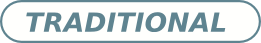# Green's functions technique for calculating the emission spectrum in a quantum dot-cavity system

• Edgar Arturo Gómez Universidad del Quindío
• José David Hernández
Keywords: microcavity, master equation, open quantum system, QD-Cavity

### Abstract

We introduce the Green's functions technique as an alternative theory to the quantum regression theorem formalism for calculating the two-time correlation functions in open quantum systems at the steady state. In order to investigate the potential of this theoretical approach, we consider a dissipative system composed of a single quantum dot inside a semiconductor cavity and the emission spectrum is computed due to the quantum dot as well as the cavity. We propose an algorithm based on the Green's functions technique for computing the emission spectrum that can easily be adapted to more complex open quantum systems. We found that the numerical results based on the Green's functions technique are in perfect agreement with the quantum regression theorem formalism. Moreover, it allows overcoming the inherent theoretical difficulties associated with the direct application of the quantum regression theorem in open quantum systems.

Received: 6 September 2016,  Accepted: 5 November 2016; Edited by: J. P. Paz; DOI: http://dx.doi.org/10.4279/PIP.080008

Cite as: E A Gómez, J D Hernández-Rivero, H Vinck-Posada, Papers in Physics 8, 080008 (2016)

### Author Biography

Edgar Arturo Gómez, Universidad del Quindío
School of physicsPublished
2016-12-23
How to Cite
Gómez, E. A., Hernández, J. D., & Vinck Posada, H. (2016). Green’s functions technique for calculating the emission spectrum in a quantum dot-cavity system. Papers in Physics, 8, 080008. https://doi.org/10.4279/pip.080008
Issue
Section
Articles